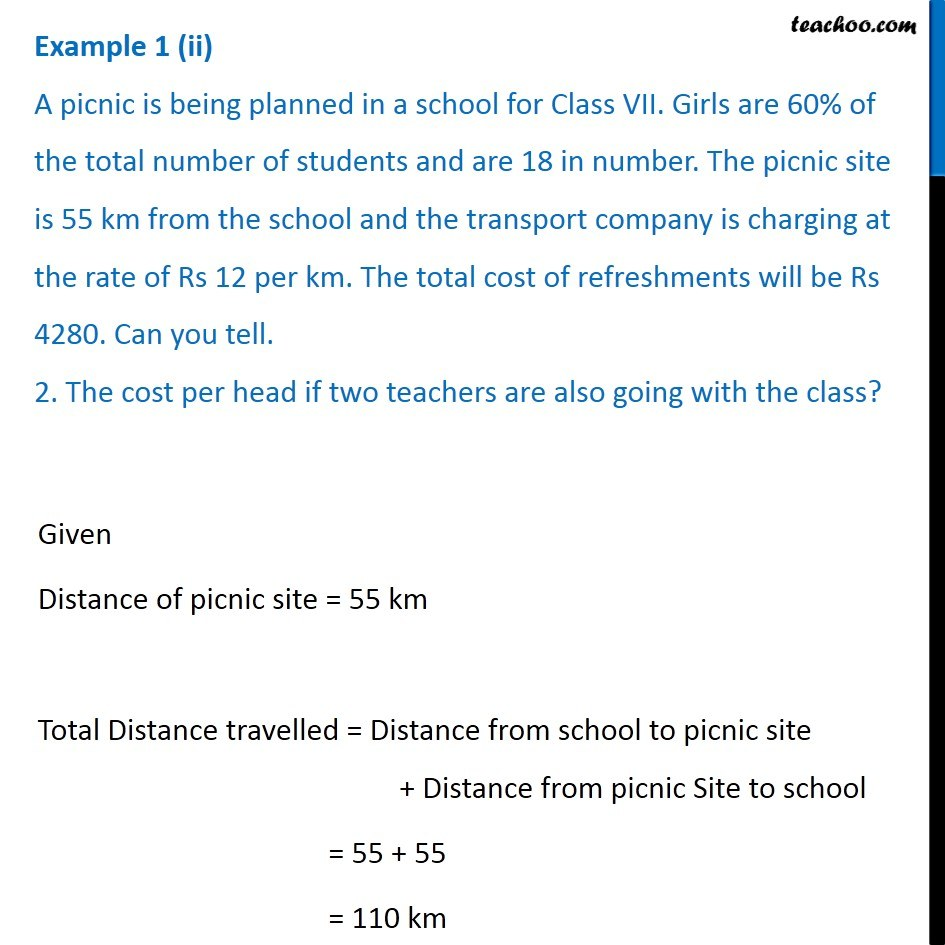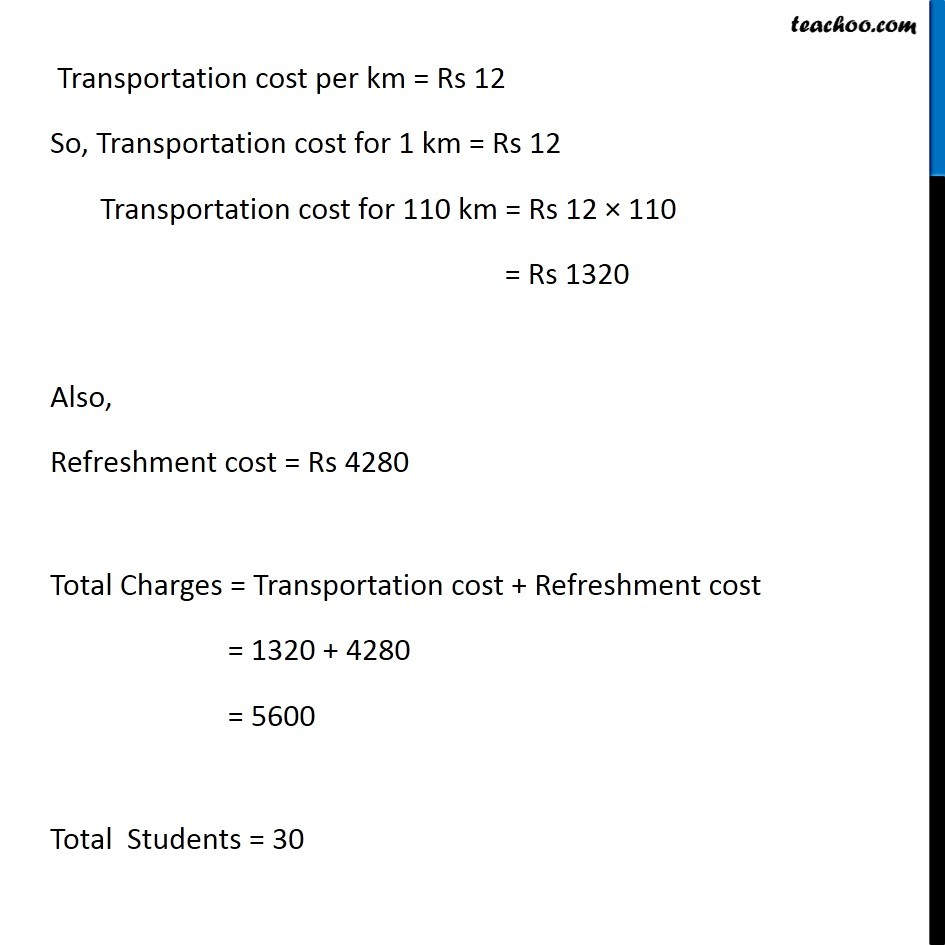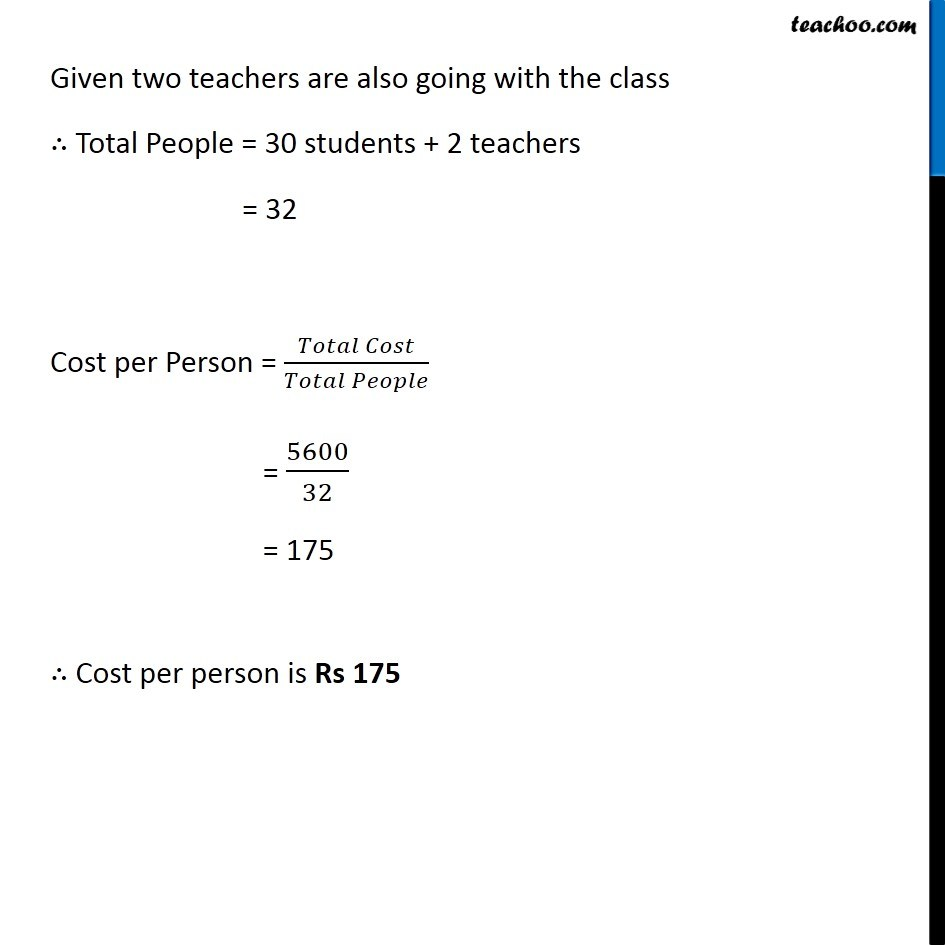Examples

Chapter 7 Class 8 Comparing Quantities
Serial order wiseLearn in your speed, with individual attention - Teachoo Maths 1-on-1 Class

### Transcript

Example 1 (ii) A picnic is being planned in a school for Class VII. Girls are 60% of the total number of students and are 18 in number. The picnic site is 55 km from the school and the transport company is charging at the rate of Rs 12 per km. The total cost of refreshments will be Rs 4280. Can you tell. 2. The cost per head if two teachers are also going with the class?Now, Cost per head = (𝑇𝑜𝑡𝑎𝑙 𝐶𝑜𝑠𝑡)/(𝑇𝑜𝑡𝑎𝑙 𝑃𝑒𝑜𝑝𝑙𝑒 𝑔𝑜𝑖𝑛𝑔) Now, Total Students = 30 Given two teachers are also going with the class ∴ Total People = 30 students + 2 teachers = 32 Now, let’s find Total cost Given that The picnic site is 55 km from the school and the transport company is charging at the rate of Rs 12 per km. The total cost of refreshments will be Rs 4280. Since Distance of picnic site = 55 km Total Distance travelled = Distance from school to picnic site + Distance from picnic Site to school = 55 + 55 = 110 km Given that Transportation cost = Rs 12 per km So, Transportation cost for 1 km = Rs 12 Transportation cost for 110 km = Rs 12 × 110 = Rs 1320 Also, Refreshment cost = Rs 4280 Total Charges = Transportation cost + Refreshment cost = 1320 + 4280 = 5600 Thus, Cost per Person = (𝑇𝑜𝑡𝑎𝑙 𝐶𝑜𝑠𝑡)/(𝑇𝑜𝑡𝑎𝑙 𝑃𝑒𝑜𝑝𝑙𝑒) = 𝟓𝟔𝟎𝟎/𝟑𝟐 = 175 ∴ Cost per person is Rs 175